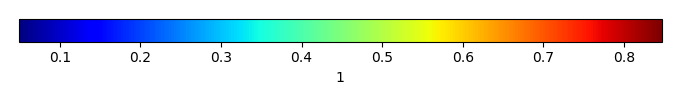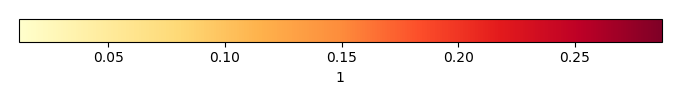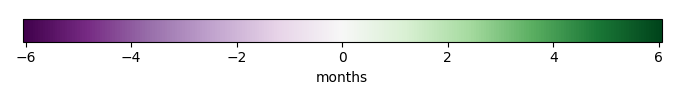# Mean State

Period Mean (original grids) 
Model Period Mean (intersection) 
Model Period Mean (complement) 
Benchmark Period Mean (intersection) 
Benchmark Period Mean (complement) 
Bias 
RMSE 
Phase Shift [months]
Bias Score 
RMSE Score 
Seasonal Cycle Score 
Spatial Distribution Score 
Interannual Variability Score 
Overall Score 
Benchmark [-] 0.138
CLM5PHSOFF [-] 0.158 0.157 0.143 0.0572 0.0141 0.0301 3.04 0.301 0.292 0.499 0.945 0.583 0.485
CLM5PHSON [-] 0.156 0.155 0.143 0.0572 0.0121 0.0287 3.03 0.323 0.307 0.505 0.943 0.546 0.488
Period Mean (original grids) 
Model Period Mean (intersection) 
Model Period Mean (complement) 
Benchmark Period Mean (intersection) 
Benchmark Period Mean (complement) 
Bias 
RMSE 
Phase Shift [months]
Bias Score 
RMSE Score 
Seasonal Cycle Score 
Spatial Distribution Score 
Interannual Variability Score 
Overall Score 
Benchmark [-] 0.181
CLM5PHSOFF [-] 0.190 0.192 0.182 0.0612 0.00871 0.0345 3.28 0.422 0.232 0.454 0.981 0.715 0.506
CLM5PHSON [-] 0.186 0.187 0.182 0.0612 0.00407 0.0324 2.99 0.433 0.264 0.507 0.973 0.647 0.515
Period Mean (original grids) 
Model Period Mean (intersection) 
Model Period Mean (complement) 
Benchmark Period Mean (intersection) 
Benchmark Period Mean (complement) 
Bias 
RMSE 
Phase Shift [months]
Bias Score 
RMSE Score 
Seasonal Cycle Score 
Spatial Distribution Score 
Interannual Variability Score 
Overall Score 
Benchmark [-] 0.142
CLM5PHSOFF [-] 0.158 0.158 0.146 0.0640 0.0117 0.0341 2.78 0.403 0.220 0.550 0.933 0.699 0.504
CLM5PHSON [-] 0.157 0.157 0.146 0.0640 0.0106 0.0316 2.50 0.399 0.266 0.602 0.910 0.626 0.511
Period Mean (original grids) 
Model Period Mean (intersection) 
Model Period Mean (complement) 
Benchmark Period Mean (intersection) 
Benchmark Period Mean (complement) 
Bias 
RMSE 
Phase Shift [months]
Bias Score 
RMSE Score 
Seasonal Cycle Score 
Spatial Distribution Score 
Interannual Variability Score 
Overall Score 
Benchmark [-] 0.368
CLM5PHSOFF [-] 0.348 0.347 0.370 0.336 -0.0238 0.136 1.29 0.754 0.589 0.855 0.980 0.750 0.753
CLM5PHSON [-] 0.323 0.322 0.370 0.336 -0.0485 0.125 1.11 0.724 0.613 0.889 0.979 0.725 0.757
Period Mean (original grids) 
Model Period Mean (intersection) 
Model Period Mean (complement) 
Benchmark Period Mean (intersection) 
Benchmark Period Mean (complement) 
Bias 
RMSE 
Phase Shift [months]
Bias Score 
RMSE Score 
Seasonal Cycle Score 
Spatial Distribution Score 
Interannual Variability Score 
Overall Score 
Benchmark [-] 0.0844
CLM5PHSOFF [-] 0.138 0.138 0.0928 0.0564 0.0451 0.0499 3.02 0.207 0.336 0.493 0.0560 0.453 0.314
CLM5PHSON [-] 0.136 0.136 0.0928 0.0564 0.0432 0.0484 3.07 0.220 0.346 0.478 0.0319 0.416 0.306
Period Mean (original grids) 
Model Period Mean (intersection) 
Model Period Mean (complement) 
Benchmark Period Mean (intersection) 
Benchmark Period Mean (complement) 
Bias 
RMSE 
Phase Shift [months]
Bias Score 
RMSE Score 
Seasonal Cycle Score 
Spatial Distribution Score 
Interannual Variability Score 
Overall Score 
Benchmark [-] 0.221
CLM5PHSOFF [-] 0.233 0.233 0.222 0.157 0.0105 0.132 1.16 0.763 0.504 0.876 0.956 0.739 0.724
CLM5PHSON [-] 0.200 0.199 0.222 0.157 -0.0233 0.0930 1.07 0.777 0.610 0.894 0.981 0.687 0.760
Period Mean (original grids) 
Model Period Mean (intersection) 
Model Period Mean (complement) 
Benchmark Period Mean (intersection) 
Benchmark Period Mean (complement) 
Bias 
RMSE 
Phase Shift [months]
Bias Score 
RMSE Score 
Seasonal Cycle Score 
Spatial Distribution Score 
Interannual Variability Score 
Overall Score 
Benchmark [-] 0.201
CLM5PHSOFF [-] 0.205 0.205 0.204 0.0927 0.000405 0.0647 1.08 0.672 0.479 0.845 0.942 0.709 0.688
CLM5PHSON [-] 0.201 0.202 0.204 0.0927 -0.00305 0.0621 1.09 0.681 0.491 0.843 0.942 0.699 0.691
Period Mean (original grids) 
Model Period Mean (intersection) 
Model Period Mean (complement) 
Benchmark Period Mean (intersection) 
Benchmark Period Mean (complement) 
Bias 
RMSE 
Phase Shift [months]
Bias Score 
RMSE Score 
Seasonal Cycle Score 
Spatial Distribution Score 
Interannual Variability Score 
Overall Score 
Benchmark [-] 0.138
CLM5PHSOFF [-] 0.159 0.159 0.144 0.0587 0.0147 0.0414 3.15 0.453 0.297 0.477 0.865 0.587 0.496
CLM5PHSON [-] 0.157 0.157 0.144 0.0587 0.0127 0.0399 3.25 0.469 0.316 0.453 0.848 0.523 0.488
Period Mean (original grids) 
Model Period Mean (intersection) 
Model Period Mean (complement) 
Benchmark Period Mean (intersection) 
Benchmark Period Mean (complement) 
Bias 
RMSE 
Phase Shift [months]
Bias Score 
RMSE Score 
Seasonal Cycle Score 
Spatial Distribution Score 
Interannual Variability Score 
Overall Score 
Benchmark [-] 0.367
CLM5PHSOFF [-] 0.347 0.344 0.366 0.392 -0.0213 0.119 1.50 0.791 0.598 0.830 0.983 0.757 0.759
CLM5PHSON [-] 0.339 0.337 0.366 0.392 -0.0292 0.111 1.40 0.787 0.617 0.849 0.983 0.762 0.769
Period Mean (original grids) 
Model Period Mean (intersection) 
Model Period Mean (complement) 
Benchmark Period Mean (intersection) 
Benchmark Period Mean (complement) 
Bias 
RMSE 
Phase Shift [months]
Bias Score 
RMSE Score 
Seasonal Cycle Score 
Spatial Distribution Score 
Interannual Variability Score 
Overall Score 
Benchmark [-] 0.153
CLM5PHSOFF [-] 0.170 0.170 0.160 0.0616 0.0105 0.0343 2.30 0.431 0.315 0.635 0.803 0.699 0.533
CLM5PHSON [-] 0.167 0.167 0.160 0.0616 0.00755 0.0333 2.40 0.443 0.326 0.618 0.781 0.680 0.529
Period Mean (original grids) 
Model Period Mean (intersection) 
Model Period Mean (complement) 
Benchmark Period Mean (intersection) 
Benchmark Period Mean (complement) 
Bias 
RMSE 
Phase Shift [months]
Bias Score 
RMSE Score 
Seasonal Cycle Score 
Spatial Distribution Score 
Interannual Variability Score 
Overall Score 
Benchmark [-] 0.146
CLM5PHSOFF [-] 0.168 0.168 0.153 0.0665 0.0154 0.0675 1.41 0.583 0.392 0.799 0.949 0.653 0.628
CLM5PHSON [-] 0.164 0.165 0.153 0.0665 0.0115 0.0634 1.43 0.578 0.405 0.796 0.912 0.667 0.627
Period Mean (original grids) 
Model Period Mean (intersection) 
Model Period Mean (complement) 
Benchmark Period Mean (intersection) 
Benchmark Period Mean (complement) 
Bias 
RMSE 
Phase Shift [months]
Bias Score 
RMSE Score 
Seasonal Cycle Score 
Spatial Distribution Score 
Interannual Variability Score 
Overall Score 
Benchmark [-] 0.147
CLM5PHSOFF [-] 0.274 0.274 0.262 0.0914 0.0118 0.0633 2.23 0.507 0.376 0.647 0.994 0.663 0.594
CLM5PHSON [-] 0.268 0.268 0.262 0.0914 0.00606 0.0584 2.21 0.510 0.398 0.648 0.993 0.640 0.598
Period Mean (original grids) 
Model Period Mean (intersection) 
Model Period Mean (complement) 
Benchmark Period Mean (intersection) 
Benchmark Period Mean (complement) 
Bias 
RMSE 
Phase Shift [months]
Bias Score 
RMSE Score 
Seasonal Cycle Score 
Spatial Distribution Score 
Interannual Variability Score 
Overall Score 
Benchmark [-] 0.284
CLM5PHSOFF [-] 0.314 0.314 0.293 0.0694 0.0211 0.0513 3.40 0.311 0.277 0.421 0.977 0.676 0.490
CLM5PHSON [-] 0.313 0.313 0.293 0.0694 0.0202 0.0516 3.39 0.311 0.276 0.423 0.978 0.674 0.490
Period Mean (original grids) 
Model Period Mean (intersection) 
Model Period Mean (complement) 
Benchmark Period Mean (intersection) 
Benchmark Period Mean (complement) 
Bias 
RMSE 
Phase Shift [months]
Bias Score 
RMSE Score 
Seasonal Cycle Score 
Spatial Distribution Score 
Interannual Variability Score 
Overall Score 
Benchmark [-] 0.399
CLM5PHSOFF [-] 0.373 0.373 0.400 0.375 -0.0269 0.118 1.27 0.783 0.620 0.840 0.993 0.738 0.766
CLM5PHSON [-] 0.363 0.363 0.400 0.375 -0.0369 0.118 1.19 0.773 0.629 0.854 0.989 0.718 0.765
Period Mean (original grids) 
Model Period Mean (intersection) 
Model Period Mean (complement) 
Benchmark Period Mean (intersection) 
Benchmark Period Mean (complement) 
Bias 
RMSE 
Phase Shift [months]
Bias Score 
RMSE Score 
Seasonal Cycle Score 
Spatial Distribution Score 
Interannual Variability Score 
Overall Score 
Benchmark [-] 0.113
CLM5PHSOFF [-] 0.150 0.150 0.125 0.0569 0.0243 0.0452 2.91 0.291 0.274 0.524 0.643 0.671 0.446
CLM5PHSON [-] 0.148 0.148 0.125 0.0569 0.0222 0.0432 3.04 0.295 0.298 0.494 0.616 0.600 0.433
Period Mean (original grids) 
Model Period Mean (intersection) 
Model Period Mean (complement) 
Benchmark Period Mean (intersection) 
Benchmark Period Mean (complement) 
Bias 
RMSE 
Phase Shift [months]
Bias Score 
RMSE Score 
Seasonal Cycle Score 
Spatial Distribution Score 
Interannual Variability Score 
Overall Score 
Benchmark [-] 0.154
CLM5PHSOFF [-] 0.166 0.165 0.159 0.0605 0.00665 0.0584 2.06 0.567 0.332 0.659 0.952 0.659 0.583
CLM5PHSON [-] 0.159 0.159 0.159 0.0605 0.000121 0.0454 2.03 0.579 0.379 0.659 0.892 0.680 0.594
Period Mean (original grids) 
Model Period Mean (intersection) 
Model Period Mean (complement) 
Benchmark Period Mean (intersection) 
Benchmark Period Mean (complement) 
Bias 
RMSE 
Phase Shift [months]
Bias Score 
RMSE Score 
Seasonal Cycle Score 
Spatial Distribution Score 
Interannual Variability Score 
Overall Score 
Benchmark [-] 0.193
CLM5PHSOFF [-] 0.200 0.200 0.197 0.0857 0.00411 0.116 0.803 0.693 0.509 0.928 0.975 0.680 0.716
CLM5PHSON [-] 0.174 0.175 0.197 0.0857 -0.0213 0.0864 0.732 0.729 0.589 0.933 0.981 0.640 0.743
Period Mean (original grids) 
Model Period Mean (intersection) 
Model Period Mean (complement) 
Benchmark Period Mean (intersection) 
Benchmark Period Mean (complement) 
Bias 
RMSE 
Phase Shift [months]
Bias Score 
RMSE Score 
Seasonal Cycle Score 
Spatial Distribution Score 
Interannual Variability Score 
Overall Score 
Benchmark [-] 0.192
CLM5PHSOFF [-] 0.186 0.186 0.194 0.0720 -0.00792 0.0616 1.35 0.663 0.406 0.800 0.986 0.699 0.660
CLM5PHSON [-] 0.177 0.177 0.194 0.0720 -0.0170 0.0587 1.51 0.622 0.428 0.771 0.976 0.708 0.655
Period Mean (original grids) 
Model Period Mean (intersection) 
Model Period Mean (complement) 
Benchmark Period Mean (intersection) 
Benchmark Period Mean (complement) 
Bias 
RMSE 
Phase Shift [months]
Bias Score 
RMSE Score 
Seasonal Cycle Score 
Spatial Distribution Score 
Interannual Variability Score 
Overall Score 
Benchmark [-] 0.134
CLM5PHSOFF [-] 0.137 0.137 0.136 0.0519 0.000703 0.0208 2.71 0.527 0.346 0.565 0.415 0.459 0.443
CLM5PHSON [-] 0.135 0.135 0.136 0.0519 -0.00112 0.0207 2.96 0.510 0.350 0.515 0.245 0.417 0.398
Period Mean (original grids) 
Model Period Mean (intersection) 
Model Period Mean (complement) 
Benchmark Period Mean (intersection) 
Benchmark Period Mean (complement) 
Bias 
RMSE 
Phase Shift [months]
Bias Score 
RMSE Score 
Seasonal Cycle Score 
Spatial Distribution Score 
Interannual Variability Score 
Overall Score 
Benchmark [-] 0.144
CLM5PHSOFF [-] 0.153 0.152 0.147 0.0557 0.00495 0.0255 3.02 0.479 0.204 0.493 0.812 0.663 0.476
CLM5PHSON [-] 0.149 0.149 0.147 0.0557 0.00174 0.0238 3.18 0.475 0.230 0.458 0.649 0.638 0.446
Period Mean (original grids) 
Model Period Mean (intersection) 
Model Period Mean (complement) 
Benchmark Period Mean (intersection) 
Benchmark Period Mean (complement) 
Bias 
RMSE 
Phase Shift [months]
Bias Score 
RMSE Score 
Seasonal Cycle Score 
Spatial Distribution Score 
Interannual Variability Score 
Overall Score 
Benchmark [-] 0.141
CLM5PHSOFF [-] 0.157 0.157 0.149 0.0759 0.00872 0.0478 2.13 0.442 0.320 0.669 0.900 0.662 0.552
CLM5PHSON [-] 0.159 0.160 0.149 0.0759 0.0109 0.0468 2.09 0.441 0.334 0.675 0.852 0.635 0.545

# Temporally integrated period mean

BENCHMARK MEANMODEL MEANBIASBIAS SCORERMSERMSE SCOREBENCHMARK INTERANNUAL VARIABILITYMODEL INTERANNUAL VARIABILITYINTERANNUAL VARIABILITY SCOREBENCHMARK MAX MONTHMODEL MAX MONTHDIFFERENCE IN MAX MONTHSEASONAL CYCLE SCORESPATIAL TAYLOR DIAGRAMMODEL COLORS# Spatially integrated regional mean

MODEL COLORSREGIONAL MEANANNUAL CYCLEMONTHLY ANOMALYANNUAL CYCLE# All Models

BenchmarkCLM5PHSOFFCLM5PHSON# Data Information

creation_date: Wed May 14 11:10:39 PDT 2014

source_file: This product is generated from monthly 1 degree CERES EBAF Radiation observations